Venus Transit 06 June 2012

To my horror when i got up at 04:49 the sky was completely clouded, and thought that this once in a lifetime opportunity had passed.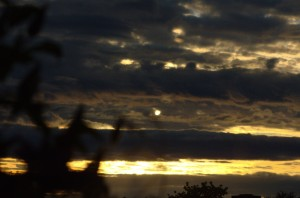Fortunately an hour later it was good enough to see the transit.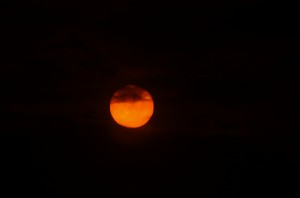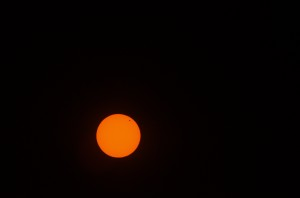And then final contact at around 06:51.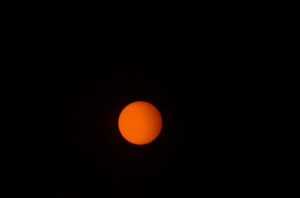The transit of venus, from sunrise, around 04:34 to last contact at around 06:50, Between 04:50 and 05:40 the sun was hidden behind the clouds, each photo is separated by 10 minutes. The posistion of the sun has been placed at the calculated position using the matlab listed script below.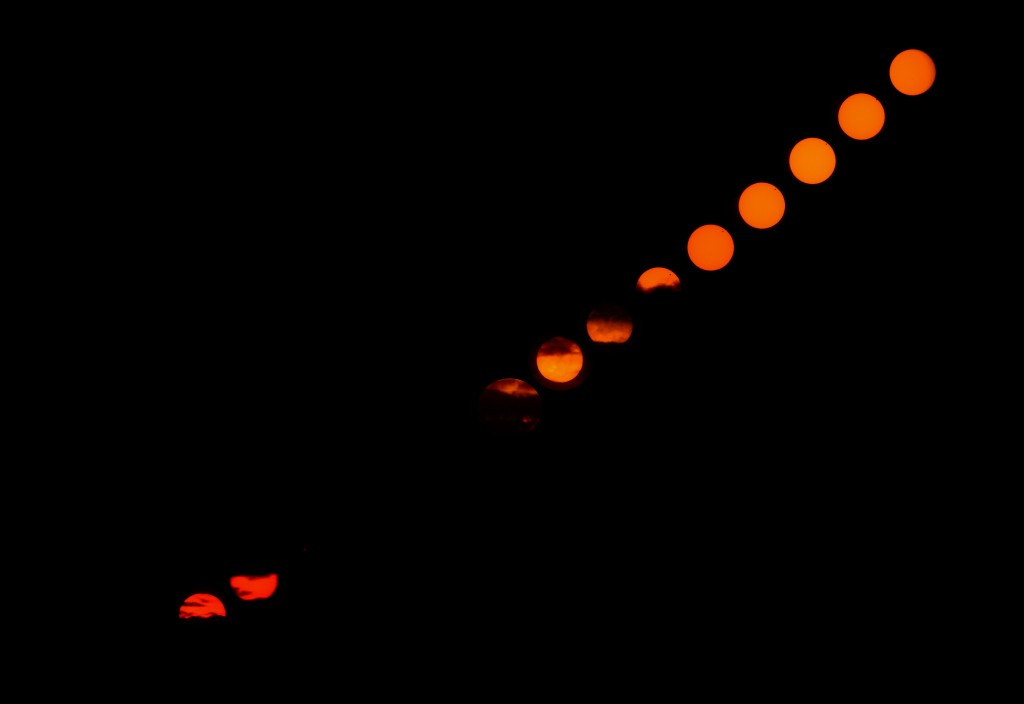This script requires the sun_position.m script from matlab central.

location.longitude = 10.7177;
location.latitude = 59.938;
location.altitude = 100;
time.year = 2012;
time.month = 6;
time.day = 06;
time.hour = 04;
time.min = 34;
time.sec = 0;
time.UTC = 2;
figure(2);
clf;
h=[];
sun_a=[];
for j=4:1:6
time.hour=j;
startt=0;
endt=59;
if j==4
startt=30;
end
if j==6
endt=50;
end
for i = startt:10:endt
time.min=i;
sun=sun_position(time,location);
sun_a=[sun_a;[j i sun.zenith sun.azimuth]];
end
end
plot(sun_a(:,4),90-sun_a(:,3),'x')
xlabel('Angle of sun (° from northl)');
ylabel('Angle of sun (° off horizontal)');
savefig(['sun_angle_venus'],figure(2),'pdf','png','-c0.1','-r300');